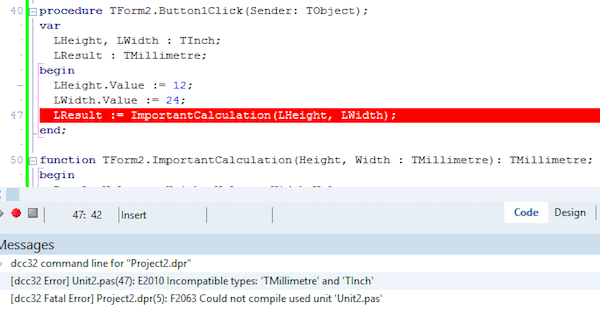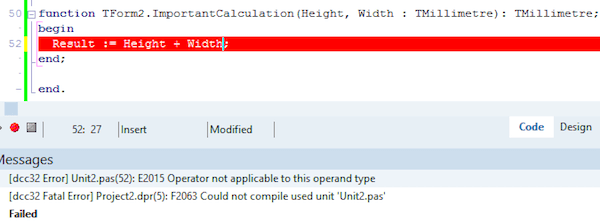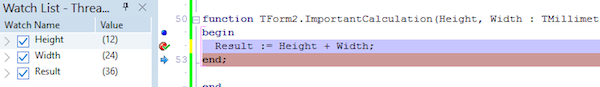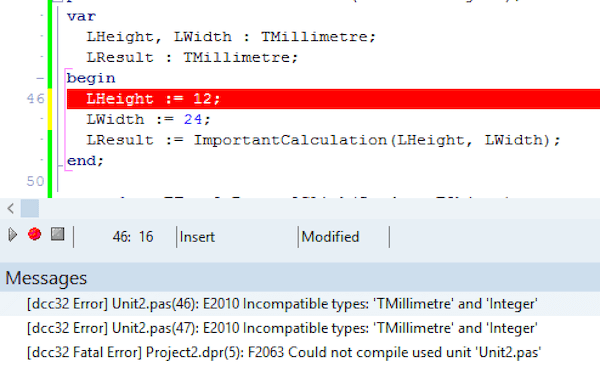In a recent Embarcadero webinar, Marco was demonstrating some new features of Records, including a new Assign operator that can be overloaded.

Afterwards I had a number of questions from people asking what it was all about. Why do we need an Assign operator? Why would you want to overload an operator anyway? After a bit of a discussion with them, I realised that rather than me trying to throw off a couple of sentences to explain it, I should probably write up something clearer.

I should note that Operator Overloading for Records has been in Delphi for quite awhile (Delphi 2010 I think). If you’ve got a relatively recent version of Delphi you can do everything I do in this article.

## The Angry Inch

So let’s start with a problem. We’re working with distance measurements in an application. Some of them are metric (eg. millimetres, centimetres and metres) and some are Imperial (Inches, Feet, Yards and other things my Dad understands but I don’t). In order to avoid a Mars Climate Orbiter style mistake, we’re going to have to be extra careful not to do things like this in our code:

```procedure TForm2.Button1Click(Sender: TObject);
var
LHeightInInches, LWidthInInches : Integer;
LResultInMillimetres : Integer;
begin
LHeightInInches := 12;
LWidthInInches := 24;
LResultInMillimetres := ImportantCalculation(LHeightInInches, LWidthInInches);
end;

function TForm2.ImportantCalculation(Height, Width: Integer): Integer;
begin
Result := Height + Width;
end;
```

This is an extremely simplified example, on purpose, but imagine some of this code is off inside methods of a class and some is calling code in another class. Even though I’ve named the variables to indicate their unit, just to try and make it obvious, it’s still going to be pretty easy to pass in a value in the wrong unit.

What I’d really like is for the Delphi compiler to check the units for me, and throw a compiler error if I mix them inappropriately. But I still want to keep the nice, clear syntax in the calculation above.

Well, let’s see what we can do. We can start by defining a record to represent Millimetres and another to represent Inches:

```TMillimetre = record
Value : Extended;
end;

TInch = record
Value : Extended;
end;
```

OK, now if I substitute those for the Integers in my example code, I get this:

```procedure TForm2.Button1Click(Sender: TObject);
var
LHeight, LWidth : TInch;
LResult : TMillimetre;
begin
LHeight.Value := 12;
LWidth.Value := 24;
LResult := ImportantCalculation(LHeight, LWidth);
end;

function TForm2.ImportantCalculation(Height, Width : TMillimetre): TMillimetre;
begin
Result.Value := Height.Value + Width.Value;
end;
```

Hmmm, two steps forward, possibly 3 or 4 steps back.

Yes, we’ve achieved one goal of getting the compiler to spot our error:but the price we’ve paid is to lose some of the clarity of our calculations and assignments. All those .Value’s scattered around the place are pretty ugly.

Let’s fix our compiler error by changing our TInch variables to TMillimetre and see what we can do to get some of our clarity back.

First off, let’s focus on this line:

```  Result.Value := Height.Value + Width.Value;
```

Why can’t we just remove the .Value calls? If we try it, the compiler will throw an “Operator not applicable to this operand type” error. The operator in question is the + operator.Why? Because it doesn’t know how to add two TMillimetre records together. Integers, Doubles, even strings, no problem, but not Records. So we need to teach it how, and this is where Operator Overloading comes in.

We need to overload the Add operator on our TMillimetre so that it knows how to add itself to another TMillimetre.

Let’s extend our TMillimetre declaration like this:

```TMillimetre = record
Value : Extended;
class operator Add(const ALeft, ARight: TMillimetre) : TMillimetre;
end;

...

class operator TMillimetre.Add(const ALeft, ARight: TMillimetre): TMillimetre;
begin
Result.Value := ALeft.Value + ARight.Value;
end;```

It looks like a function, but it’s an operator instead. When the compiler sees we’re trying to add two TMillimetre records together, something it doesn’t know how to do, it will call this method for us instead. In the implementation we just need to do whatever is necessary to add those records and return another TMillimetre. However, be careful to return a new TMillimetre and not to change either of the records passed in. After all, it would be a little odd if a := b + c ended up changing b and/or c in the process.

Now we’ve taught the compiler how to add two TMillimetre records, we can change our ImportantCalculation back to what it looked like originally without all the .Value’s dangling around and compile happily:Add isn’t all we’d like to do of course, we might like to subtract, multiply divide, etc, so there are operators for all those as well. There’s a full list in the docs.

## All Animals Are Equal (but some are more equal than others)

Another important one to think about is the equality operator. If we write:

```var
LHeight, LWidth : TMillimetre;
LSquare : Boolean;
begin
LWidth.Value := 1200;
LHeight.Value := 1200;
if LWidth = LHeight then
LSquare := True;
end;

```

our intent seems reasonably clear to us. Once again however, the compiler will complain that the = operator is not applicable. It doesn’t know how to compare two TMillimetres. So again, we have to teach it by overriding the Equals operator:

```TMillimetre = record
Value : Extended;
class operator Equal(const ALeft, ARight: TMillimetre): Boolean;
class operator Add(const ALeft, ARight: TMillimetre) : TMillimetre;
end;

...

class operator TMillimetre.Equal(const ALeft, ARight: TMillimetre): Boolean;
begin
Result := SameValue(ALeft.Value, ARight.Value, 0.01);
end;```

Now when the compiler sees an attempt to compare two TMillimetre records with the = operator, it’ll call our Equals method instead.

Why am I using the SameValue function from System.Math instead of just comparing the two .Values? Because I’m storing the Values as floating point numbers, and so I want to do a “close enough” comparison. Check out this page in the docs for more details.

This business of  teaching the compiler new tricks is kind of cool. But let’s not lose sight of my original goal. I wanted to get back to the original clarity of my code while still adding strong typing. One place it’s still messier than the original is where I’m assigning the values to my TMillimetre records. I still have those dangling .Value’s:

```var
LHeight, LWidth : TMillimetre;
LResult : TMillimetre;
begin
LHeight.Value := 12;
LWidth.Value := 24;
LResult := ImportantCalculation(LHeight, LWidth);
end;
```

I’d like to get rid of them, but if I do, the compiler grumbles yet again:This time, it’s saying it doesn’t know how to convert between 12 and a TMillimetre.

## A Change Is Gonna Come

If you look through that list of Operators I linked to before, you’ll see two in there with weird names: Implicit and Explicit. These operators let you teach the compiler what to do when something is cast to or from our record.

In our example from above, here’s what an Explicit and an Implicit cast look like:

```var
LHeight, LWidth : TMillimetre;
LResult : TMillimetre;
begin
LHeight := TMillimetre(12); // Explicit cast
LWidth := 24; // Implicit cast
LResult := ImportantCalculation(LHeight, LWidth);
end;```

In my experience, overloading the Implicit operator also allows Explicit casts to take place, so generally I only overload Explicit when I’m not overloading Implicit. ie. I want to allow Explicit casts but not Implicit casts.

So, let’s look at our Implicit operator on TMillimetre:

```TMillimetre = record
Value : Extended;
class operator Equal(const ALeft, ARight: TMillimetre): Boolean;
class operator Add(const ALeft, ARight: TMillimetre) : TMillimetre;
class operator Implicit(const Value: Extended): TMillimetre;
end;

...

class operator TMillimetre.Implicit(const Value: Extended): TMillimetre;
begin
Result.Value := Value;
end;

```

Because this one takes an Extended as a param and returns a TMillimetre, this will allow casts from Extended to TMillimetre. If we want to be able to cast the other way as well, from a TMillimetre to an Extended, we could add another one like this:

```TMillimetre = record
Value : Extended;
class operator Equal(const ALeft, ARight: TMillimetre): Boolean;
class operator Add(const ALeft, ARight: TMillimetre) : TMillimetre;
class operator Implicit(const Value: Extended): TMillimetre;
class operator Implicit(const Value: TMillimetre): Extended;
end;

...

class operator TMillimetre.Implicit(const Value: TMillimetre): Extended;
begin
Result := Value.Value;
end;
```

Now with that last trick we’ve taught the compiler, we can finally get back to my original goal. We can write relatively clear code without a mess of .Value calls dotted all around the place, but we still have the compiler there making sure we’re only using compatible types.

```var
LHeight, LWidth : TMillimetre;
LResult : TMillimetre;
begin
LHeight := 12;
LWidth := 24;
LResult := ImportantCalculation(LHeight, LWidth);
end;

function TForm2.ImportantCalculation(Height, Width : TMillimetre): TMillimetre;
begin
Result := Height + Width;
end;
```

Whether or not you want to write code like this is not the point. Hopefully this has helped you understand what operator overloading is,  and one example of the kinds of things you can use it for.

1. My BigInteger, BigDecimal, BigRational and Decimal types all nicely demonstrate how you can use operator overloading. BigIntegers would be much less intuitively usable without it. You could use Java style, e.g. `X := a.Multiply(b).Add(c);`, but IMO, `x := a * b + c;` is easier to read and understand. FWIW, it is not sure if the Assign operator will be in the next release.
2. FWIW, instead of `class operator Add(ALeft, ARight: TMillimetre) : TMillimetre;` I would rather use `const`, i.e. `class operator Add(const ALeft, ARight: TMillimetre) : TMillimetre;`. That gives you more efficient code generation.